# Adding and Subtracting Mixed Numbers with Like DenominatorsTimes up!
Times up! You may finish answering the current question and then proceed to your score!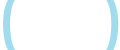Sorry, you have used all of your available hints for this lesson.
Practice Limit Reached

You've reached your daily practice limit of 12 questions.

Award InformationAwarded toQuestion HintChoose Family Member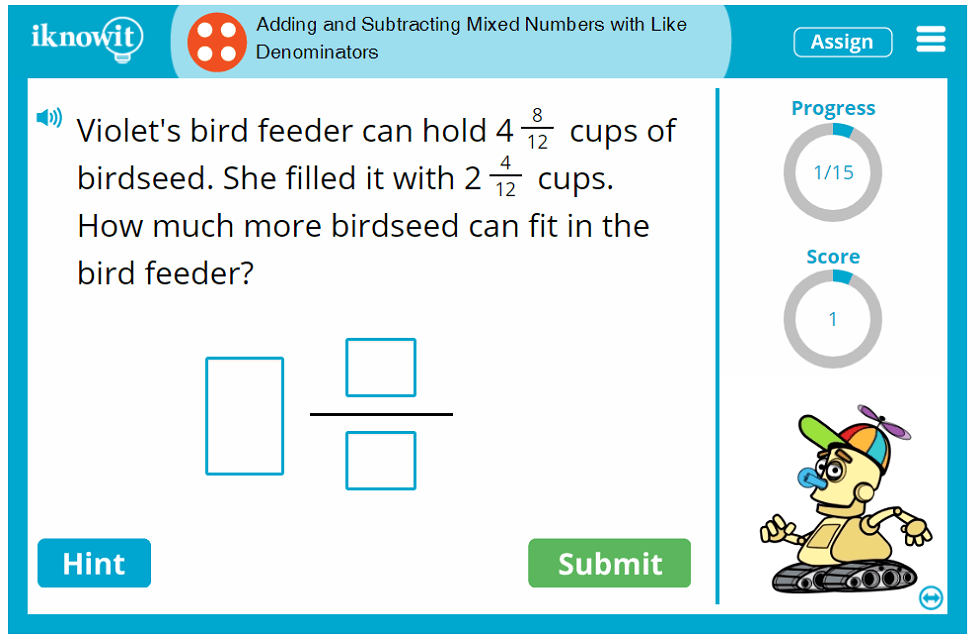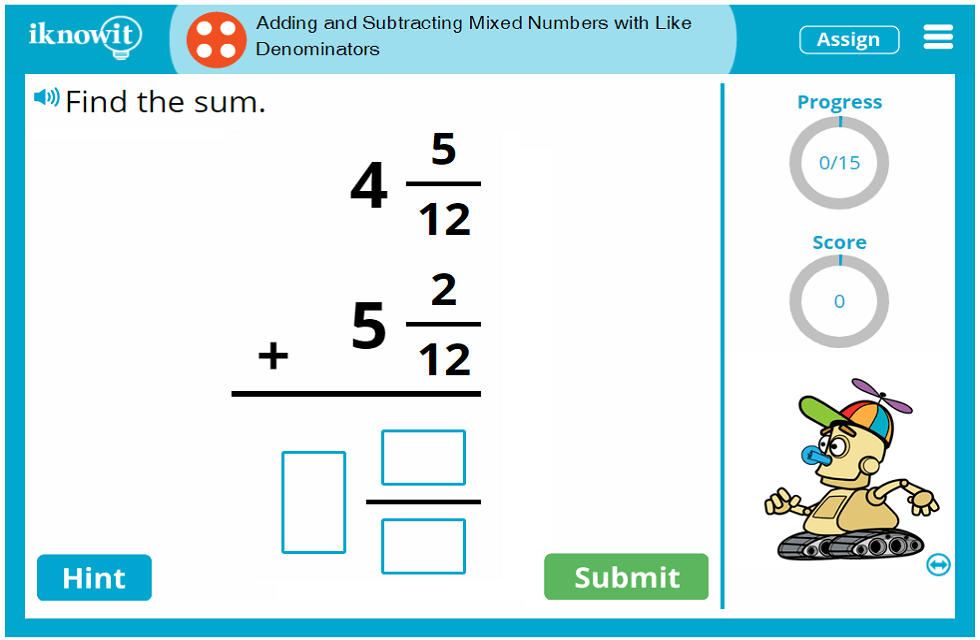## Digital Math Practice - Adding and Subtracting Mixed Numbers with Like Denominators

Get your fourth grade class geared up to practice operations with fractions with a fun digital math game! Students will put their addition and subtraction skills to the test in this interactive math lesson featuring mixed numbers with like denominators. Here are the learning objectives for this fourth grade mixed fractions activity:

• Add mixed numbers with like denominators
• Subtract mixed numbers with like denominators
• Solve mixed number addition word problems
• Solve horizontal mixed number addition and subtraction problems
• Solve vertical mixed number addition and subtraction problems

Students will use their knowledge of addition and subtraction to solve math problems involving mixed fractions and simplify if possible.

Here are a few math lesson components that are designed to help children maximize each math practice session:

• Digital math progress tracker – This tool allows students to see how many questions they have answered so far out of the total number of questions in the math lesson. Students can find the math progress tracker in the upper-right corner of their practice screen.
• Online math score tracker – This feature gives students a peek into how many points they have earned for correct answers so far in the math lesson. Students can find the math score tracker directly beneath the progress tool on their practice screen.
• Read-aloud feature – This handy option is indicated by a speaker icon in the upper-left corner of the practice screen. Students can click the button to have the question read out loud to them in a clear voice. This tool is an excellent resource for ESL/ELL students and children who are auditory processors.
• Hint button – A helpful hint is available to students when they get stuck on a mixed fractions addition or subtraction problem. Students can click the "Hint" button to view a written or pictorial clue, which may appear as a prompt for the first couple of steps in the math problem. For example: "Step 1: Add the fractional parts. Step 2: Add the whole numbers. Simplify if possible." Students can use this information to solve the problem without receiving an easy solution.
• Explanation page – When students get a n answer wrong, a detailed explanation page will pop up on the screen to show them the correct answer with an easy-to-follow explanation. Students can learn from mistakes and improve their accuracy as they move through their digital mixed numbers practice.

As these features demonstrate, iKnowIt.com math activities are designed with your students' math success in mind.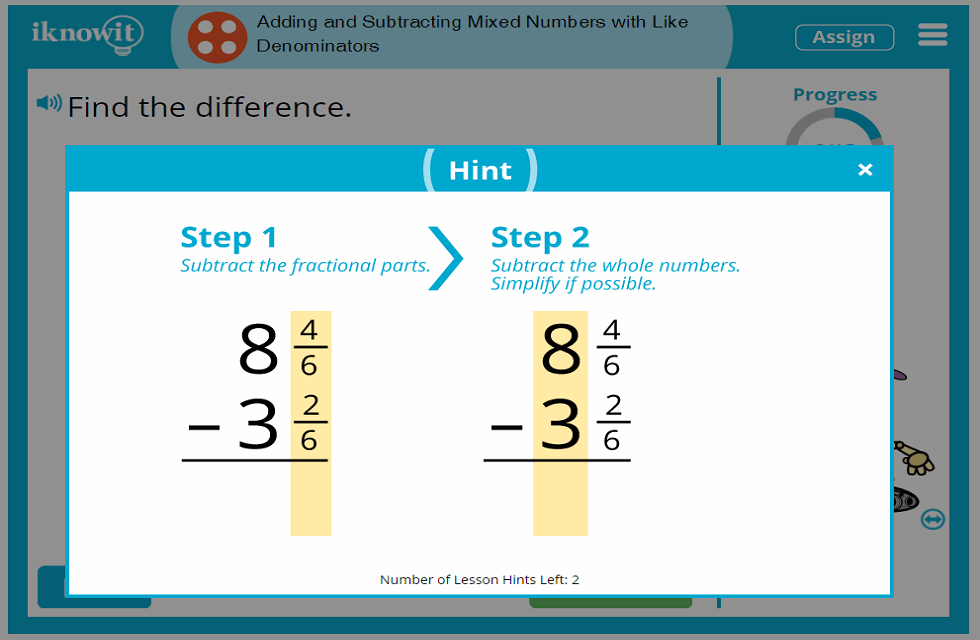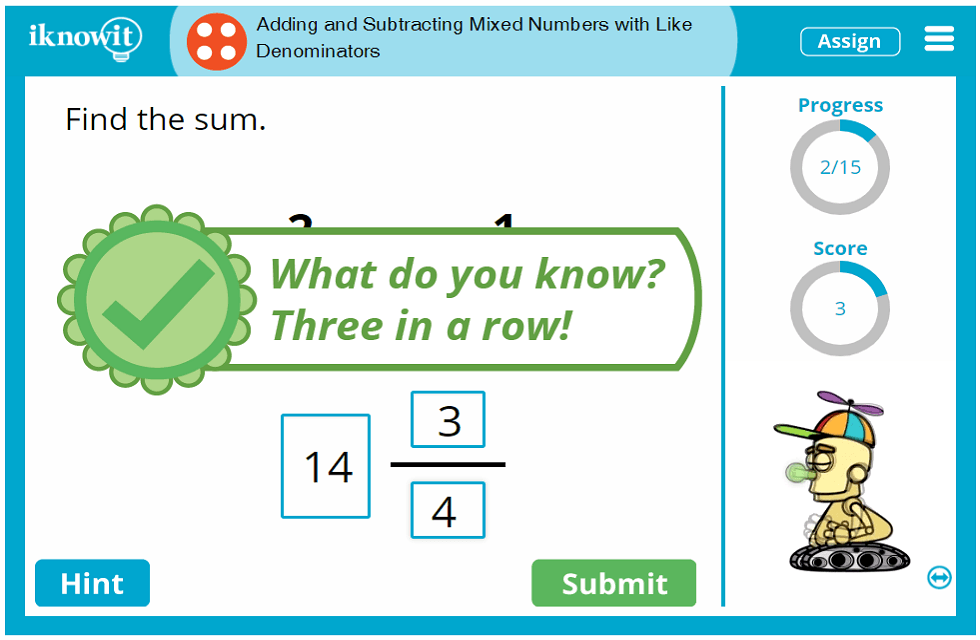## So Much More Than Online Mixed Number Practice

The I Know It program offers so much more than ordinary online math practice. Our program equips elementary teachers and homeschool educators with a math practice experience that will challenge and immerse students in math practice that is both fun and educational. Teachers love using I Know It math practice activities alongside a comprehensive elementary math curriculum to reinforce foundational math concepts, including addition and subtraction with mixed numbers. Browse dozens of math activities spanning kindergarten through fifth grade, organized on the website by grade level and topic, and aligned to the Common Core Standard. Each math lesson is written by accredited elementary math teachers and is designed to present core math topics in a kid-friendly format that is on-target with educational standards.

Your students will enjoy practicing mixed number addition and subtraction with like denominators on iKnowIt.com! Children love the bright, inviting math lesson format, complete with age-appropriate emojis and cute animated characters that dance around when they answer a question correctly. Plenty of positive feedback messages encourage little ones to "Keep going!" even when they make mistakes. Plus, students can earn math awards for completing math milestones, so you can celebrate their accomplishments together as a class.

We hope you and your fourth-grade class will enjoy practicing mixed number addition and subtraction with like denominators in this interactive math activity! Be sure to check out the hundreds of fourth grade math lessons we have available on our website as well.

• Creating a class roster for your students
• Changing basic lesson settings, like turning the animated characters on or off
• Viewing detailed class statistics and individual student progress reports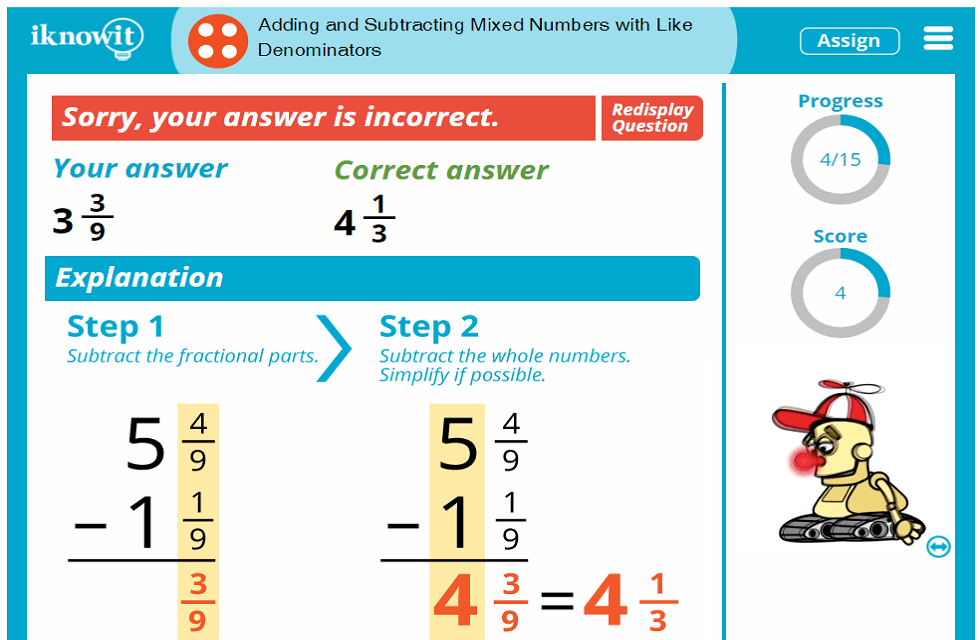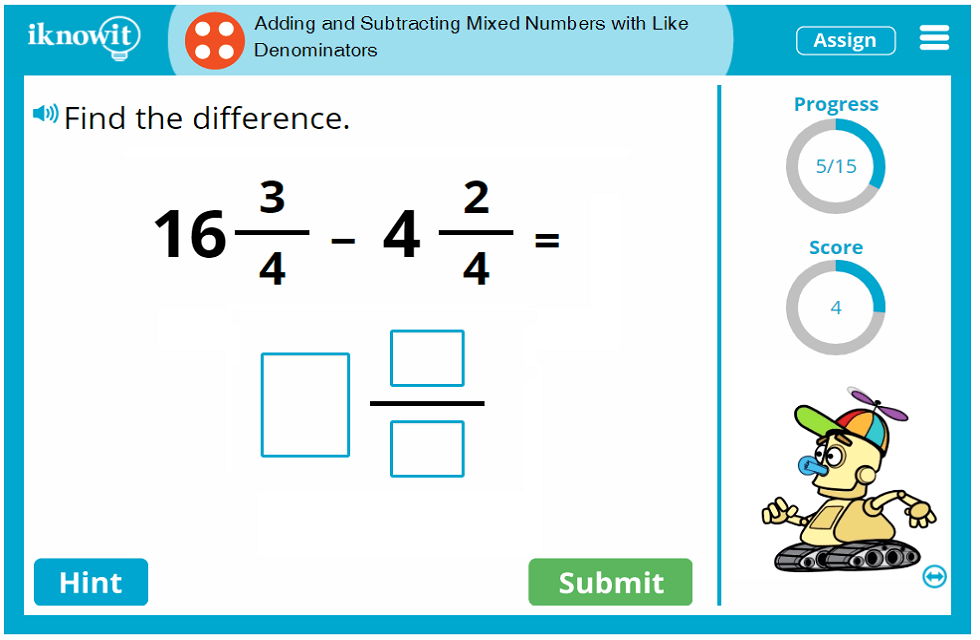## Level

This online math lesson is classified as Level D. It may be ideal for a fourth-grade class.

## Common Core Standard

4.NF.3
Numbers and Operations - Fractions

Students will build fractions from unit fractions by applying and extending previous understandings of operations on whole numbers.

## You might also be interested in...

Adding Fractions with Unlike Denominators (Level D)
In this fourth grade-level math lesson, students will practice adding fractions with unlike denominators. Questions are presented in fill-in-the-blank format.

Subtracting Fractions with Unlike Denominators (Level D)
In this math lesson geared toward fourth-grade, students will practice subtracting fractions with unlike denominators. Questions are presented in fill-in-the-blank format.